﻿ Weighted Quasi Lindley Distribution with Properties and ApplicationsInternational Journal of Statistics and Applications

p-ISSN: 2168-5193    e-ISSN: 2168-5215

2019;  9(1): 8-20

doi:10.5923/j.statistics.20190901.02### Weighted Quasi Lindley Distribution with Properties and Applications

Rama Shanker1, Kamlesh Kumar Shukla1, Tekie Asehun Leonida2

1Department of Statistics, College of Science, Eritrea Institute of Technology, Asmara, Eritrea

2Department of Applied Mathematics, University of Twente, The Netherlands

Correspondence to: Rama Shanker, Department of Statistics, College of Science, Eritrea Institute of Technology, Asmara, Eritrea.
 Email: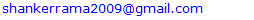Abstract

In this paper a three-parameter weighted quasi Lindley distribution which includes two-parameter quasi Lindley distribution, weighted Lindley distribution and gamma distribution and one parameter Lindley and exponential distributions as particular cases has been proposed and its statistical properties including moments and moments based measures, hazard rate function, and mean residual life function have been discussed. Maximum likelihood estimation has been discussed for estimating its parameters. Applications of the distribution have explained with two real failure time data and the goodness of fit shows quite satisfactory fit over other lifetime distributions.

Keywords: Weighted distribution, Quasi Lindley distribution, Moments, Hazard rate function, Mean residual life function, Maximum Likelihood estimation, Lifetime data, Goodness of fit

Cite this paper: Rama Shanker, Kamlesh Kumar Shukla, Tekie Asehun Leonida, Weighted Quasi Lindley Distribution with Properties and Applications, International Journal of Statistics and Applications, Vol. 9 No. 1, 2019, pp. 8-20. doi: 10.5923/j.statistics.20190901.02.

### 1. Introduction

The study of weighted distributions are useful in distribution theory because it provides a new understanding of the existing standard probability distributions and it provides methods for extending existing standard probability distributions for modeling lifetime data due to the introduction of additional parameter in the model which creates flexibility in their nature. Weighted distributions occur in modeling clustered sampling, heterogeneity, and extraneous variation in the dataset.
The concept of weighted distributions were firstly introduced by Fisher (1934) to model ascertainment biases which were later formulized by Rao (1965) in a unifying theory for problems where the observations fall in non-experimental, non-replicated and non-random manner. When observations are recorded by an investigator in the nature according to certain stochastic model, the distribution of the recorded observations will not have the original distribution unless every observation is given an equal chance of being recorded. For instance, let the original observation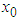comes from a distribution having probability density function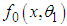, where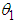may be a parameter vector and the observation x is recorded according to a probability re-weighted by weight function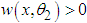,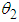being a new parameter vector, then x comes from a distribution having pdf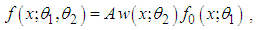(1.1)
where A is a normalizing constant. Note that such types of distribution are known as weighted distributions. The weighted distributions with weight function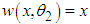are called length biased distributions or simple size-biased distributions. Patil and Rao (1977, 1978) have examined some general probability models leading to weighted probability distributions, discussed their applications and showed the occurrence of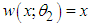in a natural way in problems relating to sampling.
Shanker and Mishra (2013) introduced a two-parameter quasi Lindley distribution (QLD) having parameters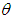and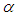and defined by its probability density function (pdf) and cumulative distribution function (cdf)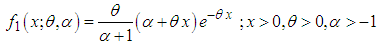(1.2)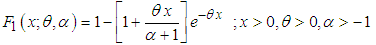(1.3)
It can be easily verified that at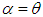, QLD reduces to Lindley distribution, introduced by Lindley (1958). Also exponential distribution is a limiting case of QLD for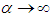. Shanker and Mishra (2016) also proposed a quasi Poisson-Lindley distribution and discussed its statistical properties, estimation of parameters and applications. Shanker et al (2016) discussed various important statistical properties and applications of QLD.
Ghitany et al (2011) introduced a two-parameter weighted Lindley distribution (WLD) with parametersanddefined by its pdf and cdf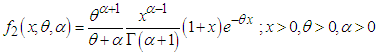(1.4)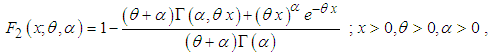(1.5)
where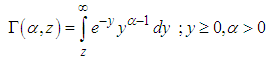(1.6)
is the upper incomplete gamma function. It can be easily verified that the Lindley distribution introduced by Lindley (1958) having pdf and cdf given by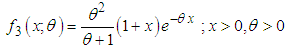(1.7)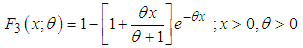(1.8)
is a particular case of (1.3) for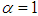. Ghitany et al (2008) have detailed study regarding its statistical and mathematical properties, estimation of parameter and application. Shanker et al (2015) have comparative study on applications of exponential and Lindley distribution for modeling lifetime data. Shanker and Shukla (2018) has obtained a Poisson mixture of weighted Lindley distribution and discussed its statistical properties, estimation of parameters and applications for discrete data.
In the present paper, a three - parameter weighted quasi Lindley distribution which includes Lindley (1958) distribution, weighted Lindley distribution and quasi Lindley distribution as particular cases, has been proposed and discussed. Its moments about origin and central moments, coefficient of variation, skewness, kurtosis and index of dispersion have been derived. The hazard rate function and the mean residual life function of the distribution have been derived and their shapes have been discussed for varying values of the parameters. The estimation of its parameters has been discussed using maximum likelihood method. Finally, the goodness of fit and the applications of the distribution have been explained through two failure time data and the fit has been compared with one parameter Lindley distribution and the two-parameter weighted Lindley distribution and quasi Lindley distribution.

### 2. Weighted Quasi Lindley Distribution

The pdf of a three-parameter weighted quasi Lindley distribution (WQLD) can be obtained as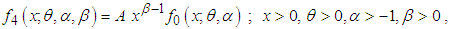(2.1)
where A is a normalizing constant and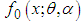is the pdf of quasi Lindley distribution introduced by Shanker and Mishra (2013) given in (1.2). Thus, the pdf of WQLD can be expressed as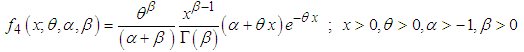(2.2)
The pdf in (2.1) can be easily expressed as a convex combination of gamma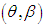and gamma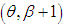distributions. We have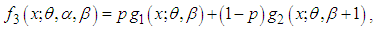(2.3)
where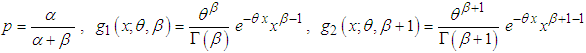.
It can be easily verified that one parameter Lindley and exponential distributions, two-parameter weighted Lindley distribution, quasi Lindley distribution and gamma distribution are particular cases of WQLD at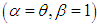,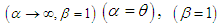and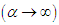, respectively.
The survival (reliability) function of WQLD can be obtained as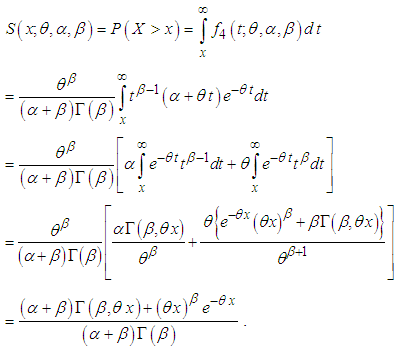where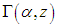is the upper incomplete gamma function defined in (1.3). The corresponding cdf of WQLD can thus be given by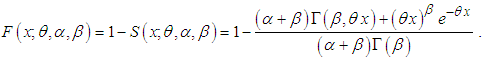The behaviour of the pdf of WQLD for varying values of parameters has been shown in figure 1. Further, the behaviour of the cdf of WQLD has also been shown in figure 2.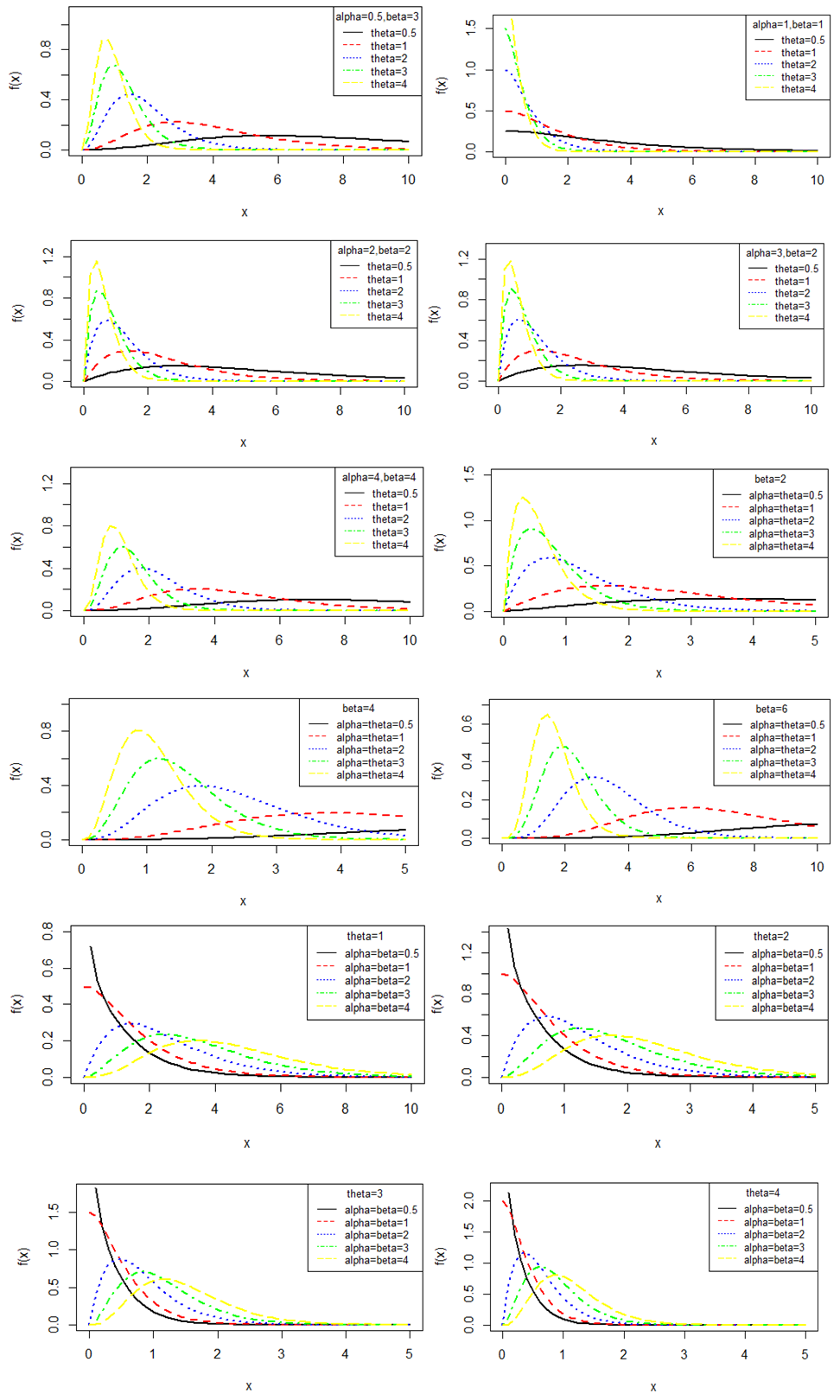Figure 1. Behaviour of the pdf of WQLD for varying values of parameters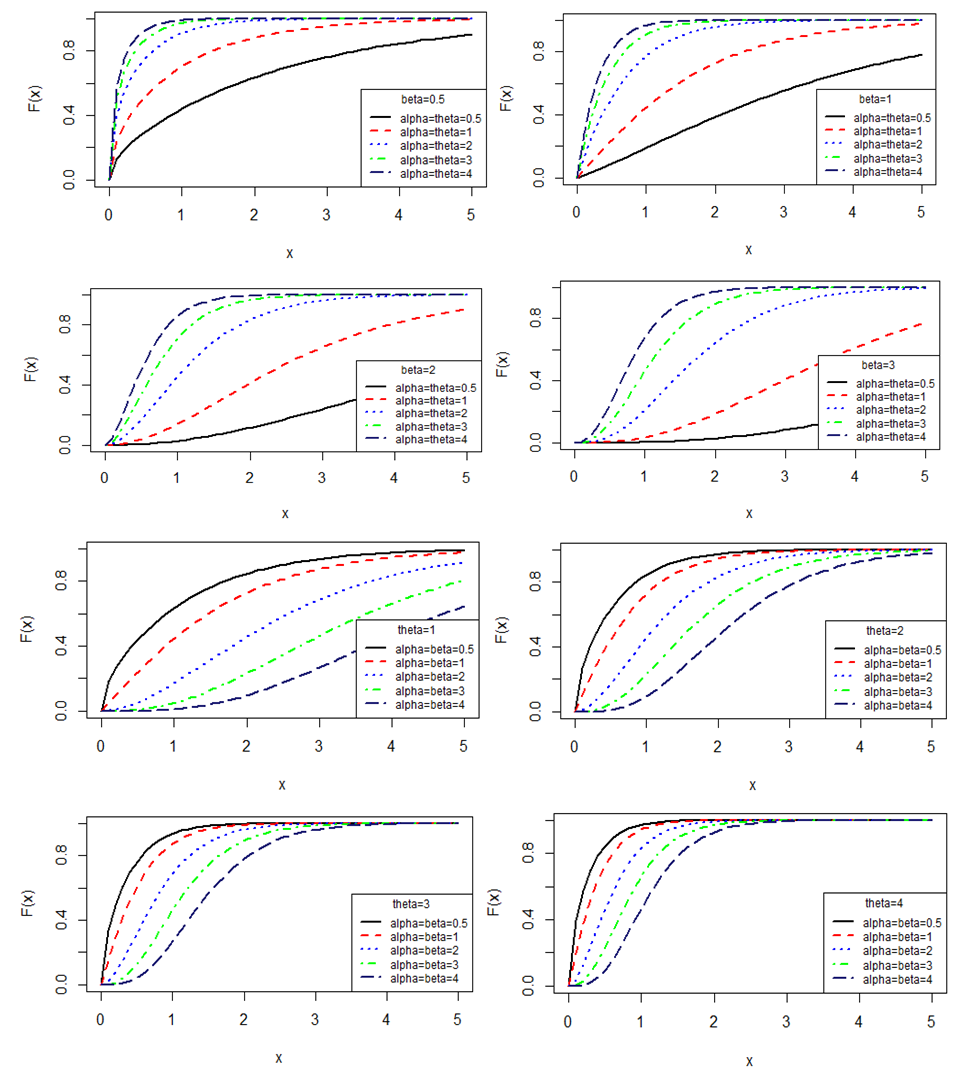Figure 2. Behaviour pf the cdf of WQLD for varying values of parameters

### 3. Statistical Characteristics

The rth moment about origin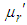of the WQLD) (2.2) can be obtained as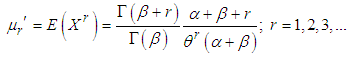(3.1)
Substituting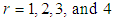in (3.1), the first four moments about origin of the WQLD are obtained as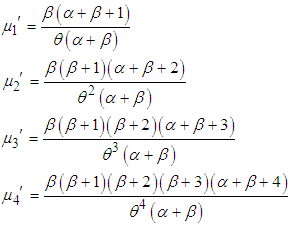Again using relationship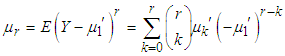between central moments and moments about origin, the central moments of WQLD are obtained as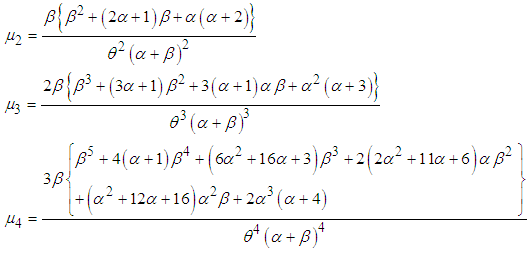The expressions for coefficient of variation (C.V.) coefficient of skewness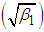, coefficient of kurtosis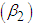, and index of dispersion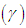of the WQLD (2.1) are thus obtained as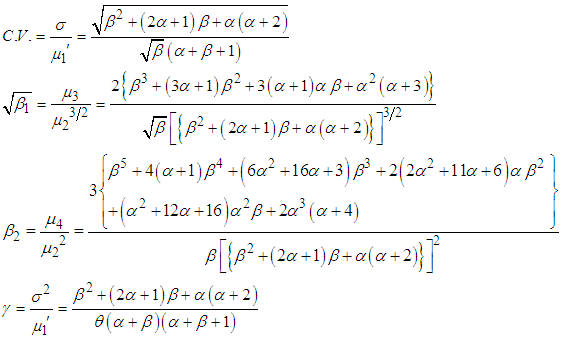The nature of the coefficient of variation, coefficient of skewness, coefficient of kurtosis and index of dispersion of WQLD for varying values of the parameters have been shown in figure 3.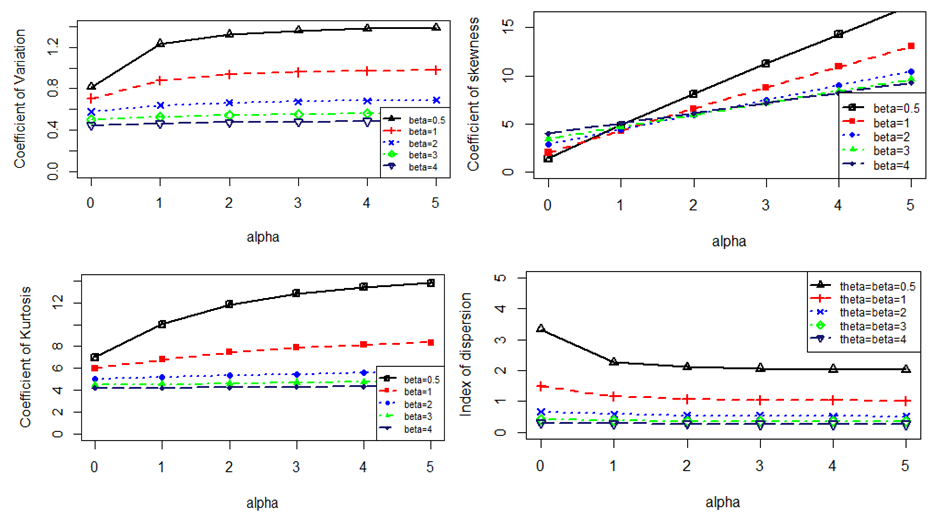Figure 3. Nature of coefficient of variation, coefficient of skewness, coefficient of kurtosis and index of dispersion of WQLD for varying values of the parameters

### 4. Reliability Measures

#### 4.1. Hazard Rate Function

The hazard (or failure) rate function,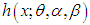of WQLD is thus obtained as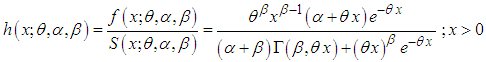The shapes of the hazard rate function,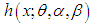of the WQLD for varying values of the parameters are shown in the figure 4.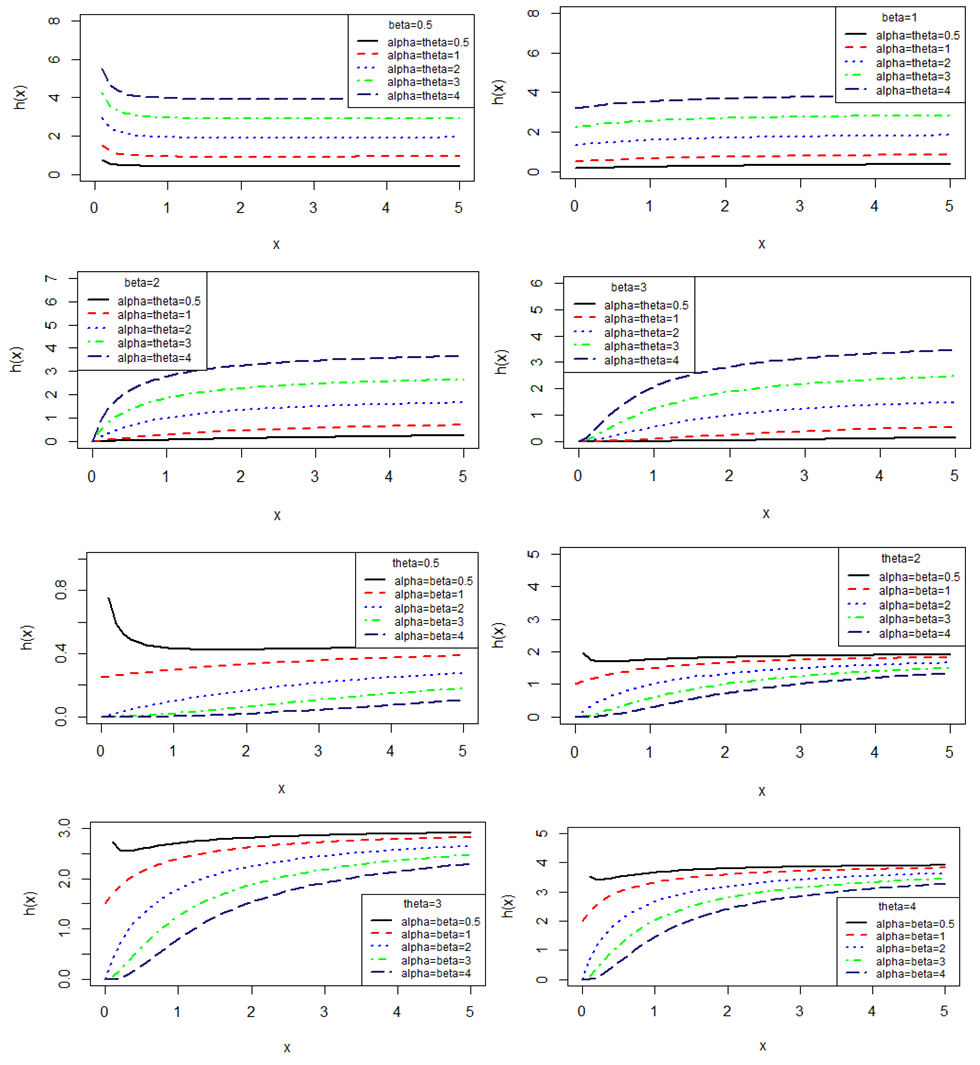Figure 4. Nature of the hazard rate function, h(x) of the WQLD for varying values of the parameters

#### 4.2. Mean Residual Life Function

The mean residual life function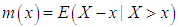of the WQLD can be obtained as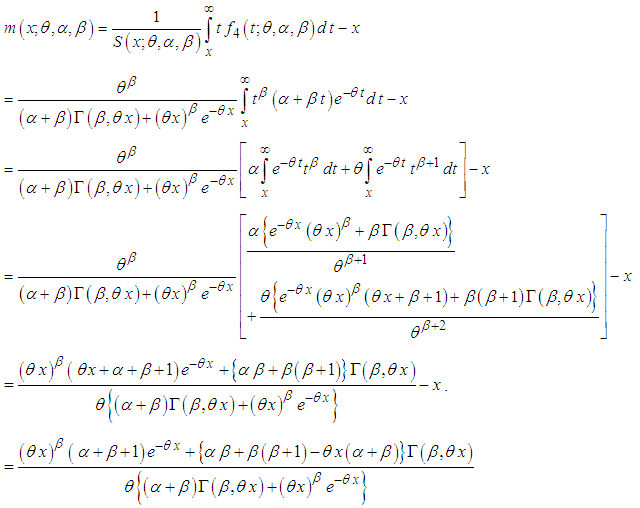The shapes of the mean residual life function,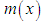of the WQLD for varying values of the parameters are shown in figure 5.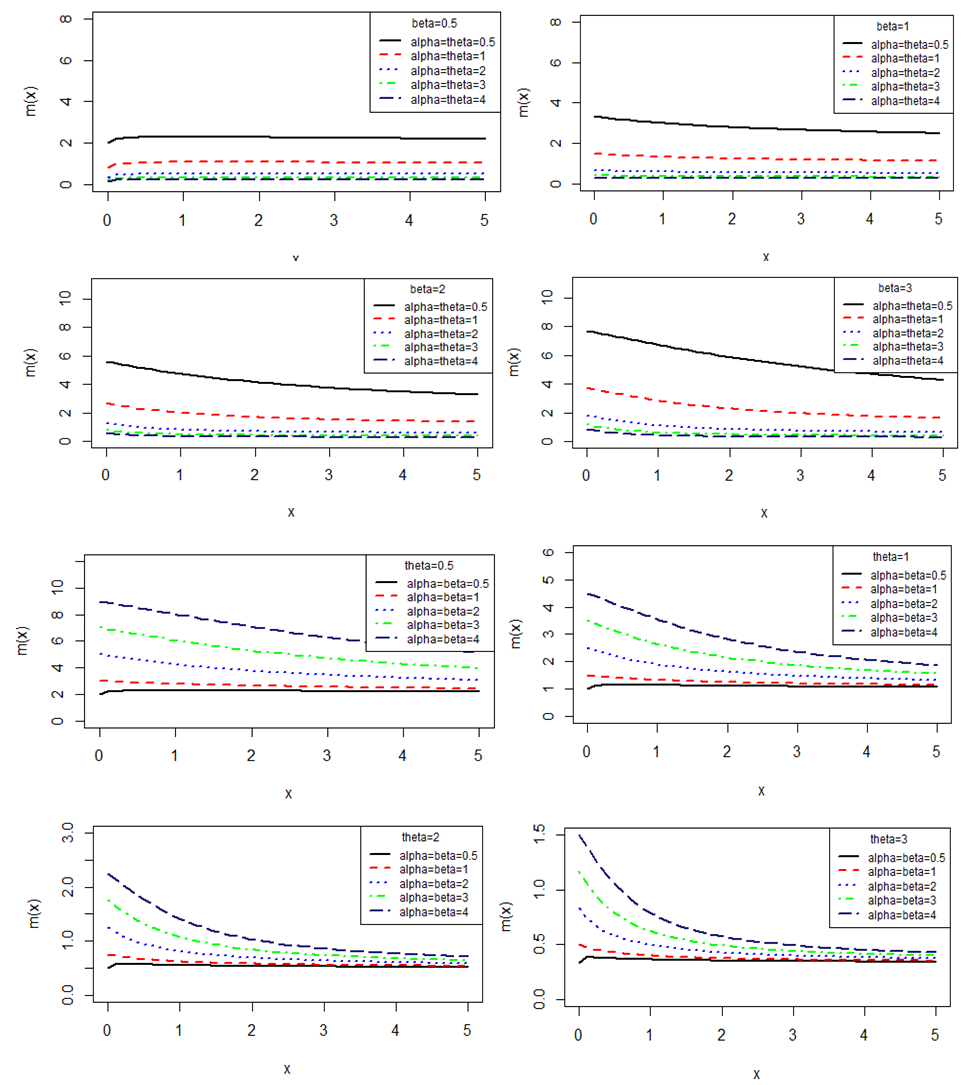Figure 5. Nature of the mean residual life function, m(x) of the WQLD for varying values of the parameters

### 5. Maximum Likelihood Estimation

Let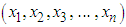be a random sample of size n from WQLD. The natural log likelihood function of WQLD can be expressed as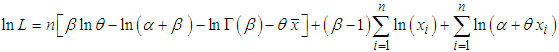The maximum likelihood estimates (MLEs)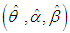of parameters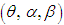of WQLD are the solution of the following nonlinear equations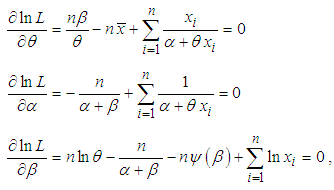where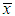is the sample mean and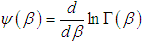is the digamma function.
These three natural log likelihood equations do not seem to be solved directly, because they cannot be expressed in closed forms. The (MLE’s)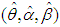of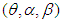can be computed directly by solving the natural log likelihood equation using Newton-Raphson iteration using R-software till sufficiently close values ofare obtained.

### 6. Applications

The applications of the WQLD have been explained with two real lifetime datasets from engineering which are available in Lawless (2003, pp. 204 and 263). The two datasets are as follows
Data Set 1: The following data set represents the failure times (in minutes) for a sample of 15 electronic components in an accelerated life test, Lawless (2003, pp. 204)
1.4 5.1 6.3 10.8 12.1 18.5 19.7 22.2 23.0 30.6 37.3 46.3 53.9 59.8 66.2
Data Set 2: The following data set represents the number of cycles to failure for 25 100-cm specimens of yarn, tested at a particular strain level, Lawless (2003, pp. 263)
15 20 38 42 61 76 86 98 121 146 149 157 175 176
180 180 198 220 224 251 264 282 321 325 653
The goodness of fit of WQLD has been compared with several distributions including generalized Lindley distribution (GLD) introduced by Zakerzadeh and Dolati (2009), generalized exponential distribution (GED) proposed by Gupta and Kundu (1999), weighted Lindley distribution (WLD) suggested by Ghitany et al (2011), Quasi Lindley distribution (QLD) proposed by Shanker and Mishra (2013), Weibull distribution introduced by Weibull (1951), gamma distribution, lognormal distribution, Lindley distribution, and exponential distribution. The pdf and the cdf of these distributions are given in table 1. The maximum likelihood estimates of parameters, standard errors of parameters,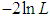, K-S (Kolmogorov-Smirnov Statistics) and p-values of the fitted distributions for datasets 1 and 2 have been shown in tables 2 and 3 respectively. The best distribution is the distribution which corresponds to the lower value ofand K-S.
It is obvious from the goodness of fit of WQLD that it gives a better fit as compared to several one parameter, two-parameter and three-parameter lifetime distributions and hence it can be considered as an important three-parameter lifetime distribution in statistics literature.
 Table 1. The pdf and the cdf of the fitted distributions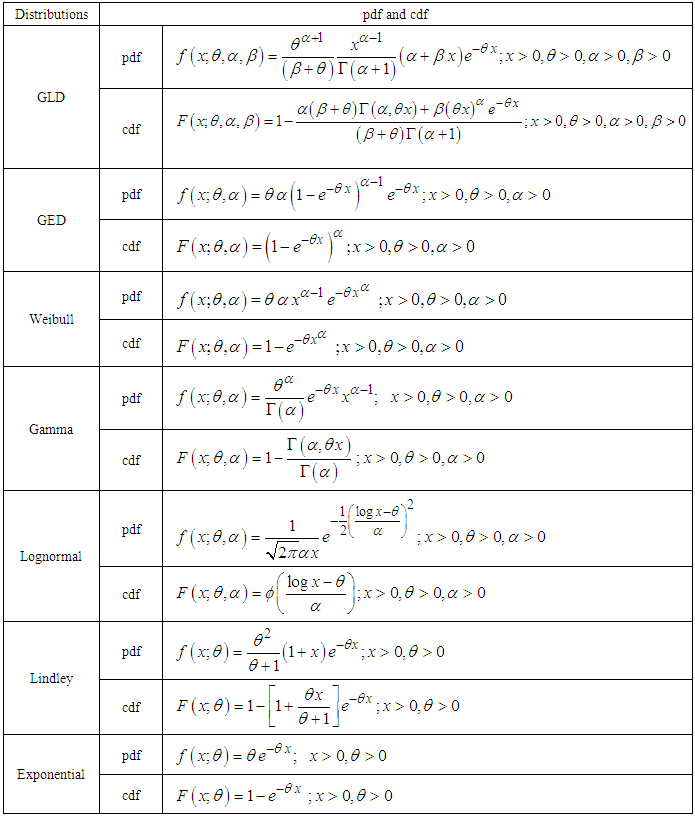Table 2. ML estimates and summary of goodness of fit for dataset 1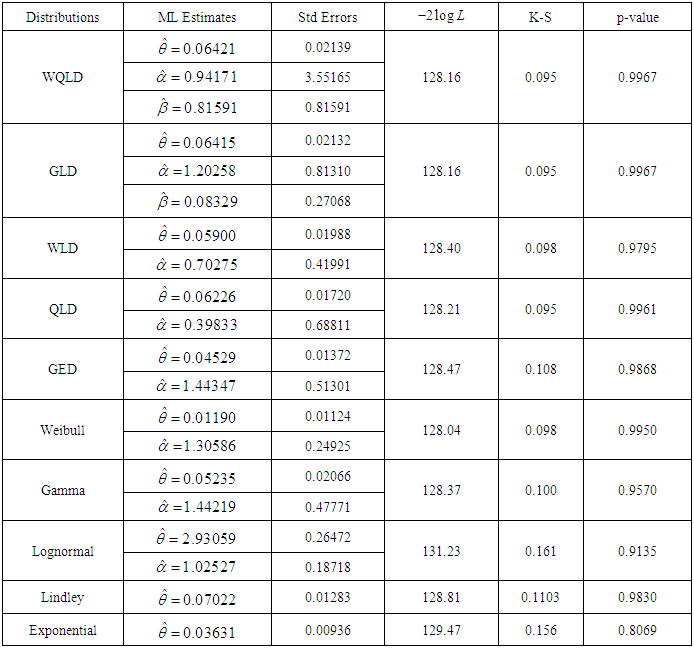Table 3. ML estimates and summary of goodness of fit for dataset 2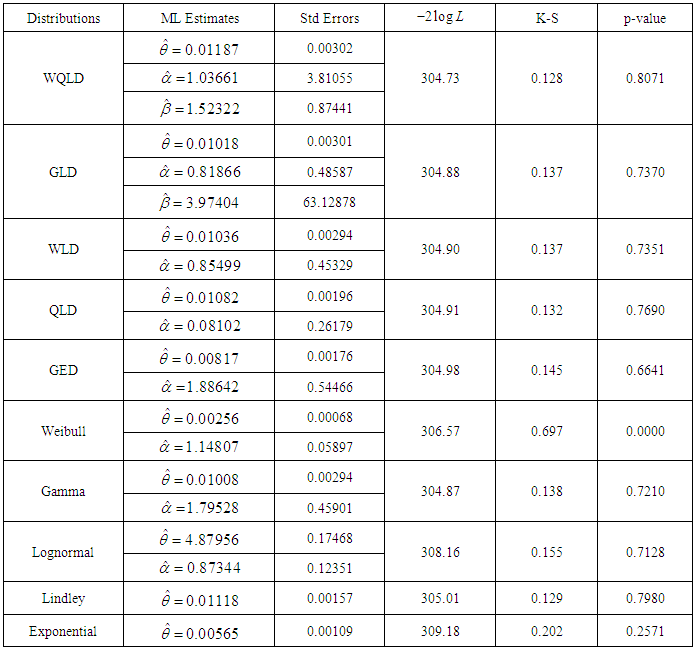### 7. Concluding Remarks

A three-parameter weighted quasi Lindley distribution (WQLD), which includes two-parameter quasi Lindley distribution, weighted Lindley distribution and gamma distribution and one parameter Lindley and exponential distributions as particular cases, has been proposed in the present paper. Some statistical properties of WQLD including moments and moments based measures, hazard rate function, and mean residual life function have been discussed. Maximum likelihood estimation has been discussed for estimating the parameters of the proposed distribution. Applications of the distribution have explained with two real failure time data.

### ACKNOWLEDGEMENTS

Authors are grateful to the editor-in-chief and the anonymous reviewer for constructive comments which enhanced the quality and the presentation of the paper.

### References

  Fisher, R.A. (1934): The effects of methods of ascertainment upon the estimation of frequencies, Ann. Eugenics, 6, 13 – 25.  Ghitany, M.E., Atieh, B. and Nadarajah, S. (2008): Lindley distribution and its Application, Mathematics Computing and Simulation, 78, 493 – 506.  Ghitany, M.E., Alqallaf, F., Al-Mutairi, D.K., Husain, H.A. (2011): A two-parameter weighted Lindley distribution and its applications to survival data, Mathematics and Computers in simulation, 81, 1190-1201.  Gupta, R.D. and Kundu, D. (1999): Generalized Exponential Distribution, Austalian & New Zealand Journal of Statistics, 41(2), 173 – 188.  Lawless, J.F. (2003): Statistical Models and Methods for Lifetime Data, Wiley, New York.  Lindley, D. V., (1958): Fiducial distributions and Bayes’ theorem, Journal of the Royal Statistical Society, Series B, 20, PP. 102- 107.  Patil, G.P. and Rao, C.R. (1977): The Weighted distributions: A survey and their applications, In applications of Statistics (Ed P.R. Krishnaiah0, 383 – 405, North Holland Publications Co., Amsterdam.  Patil, G.P. and Rao, C.R. (1978): Weighted distributions and size-biased sampling with applications to wild-life populations and human families, Biometrics, 34, 179 – 189.  Rao, C.R. (1965): On discrete distributions arising out of methods of ascertainment In: Patil, G.P.(eds) Classical and Contagious Discrete Distributions. Statistical Publishing Society, Calcutta, 320 – 332.  Shanker, R. and Mishra, A. (2013): A Quasi Lindley Distribution, African Journal of Mathematics and Computer Science Research (AJMCSR), 6 (4), 64 – 71.  Shanker, R. and Shukla, K.K. (2018): On Poisson-Weighted Lindley distribution and its Applications to appear in Journal of Scientific Research.  Shanker, R., Hagos, F. and Sujatha, S. (2015): On Modeling of Lifetimes data using Exponential and Lindley distributions, Biometrics & Biostatistics International Journal, 2(5), 1-9.  Shanker, R. and Mishra, A. (2016): A quasi Poisson-Lindley distribution, Journal of Indian Statistical Association, 54 (1&2), 113 – 125.  Shanker, R., Hagos, F. and Sharma, S. (2016): On Quasi Lindley distribution and Its Applications to Model Lifetime Data, International Journal of Statistical Distributions and Applications, 2(1), 1 – 7.  Weibull, W. (1951): A statistical distribution of wide applicability, Journal of Applied Mathematics, 18, 293 – 297.  Zakerzadeh, H. and Dolati, A. (2009): Generalized Lindley distribution, Journal of Mathematical extension, 3 (2), 13 – 25.